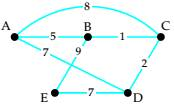# Use trial and error to find two Hamiltonian circuits of different total weights, starting at vertex A in the weighted graph. Compute the total weight of each circuit.### Mathematical Excursions (MindTap C...

4th Edition
Richard N. Aufmann + 3 others
Publisher: Cengage Learning
ISBN: 9781305965584

#### Solutions

Chapter
Section### Mathematical Excursions (MindTap C...

4th Edition
Richard N. Aufmann + 3 others
Publisher: Cengage Learning
ISBN: 9781305965584
Chapter 5.2, Problem 7ES
Textbook Problem
41 views

## Use trial and error to find two Hamiltonian circuits of different total weights, starting at vertex A in the weighted graph. Compute the total weight of each circuit.To determine

To determine two Hamiltonian circuits of different total weights, starting at vertex A and to compute the total weight of each circuit.

### Explanation of Solution

Given information:

Given, graph is:

Calculation:

A path in a Hamiltonian graph is said to be a Hamiltonian Circuit if it begins and ends at the same vertex and passes through each vertex of a graph exactly once.

A graph may contain more than one Hamiltonian circuit...

### Still sussing out bartleby?

Check out a sample textbook solution.

See a sample solution

#### The Solution to Your Study Problems

Bartleby provides explanations to thousands of textbook problems written by our experts, many with advanced degrees!

Get Started

Find more solutions based on key concepts
Convert the expressions in Exercises 8596 radical form. 45x3/2

Finite Mathematics and Applied Calculus (MindTap Course List)

Does the point P (1, 54) lie on the line 6x 8y 16 = 0? Justify your answer.

Applied Calculus for the Managerial, Life, and Social Sciences: A Brief Approach

Express the function F(x)=1/x+x as a composition of three functions.

Single Variable Calculus: Early Transcendentals, Volume I

Find the divergence of the vector field. F(x,y,z)=ln(x2+y2)i+xyj+ln(y2+z2)k.

Calculus: Early Transcendental Functions (MindTap Course List)

True or False: These lines are skew:

Study Guide for Stewart's Multivariable Calculus, 8th

The polar coordinates of the point P in the figure at the right are:

Study Guide for Stewart's Single Variable Calculus: Early Transcendentals, 8th

26. A person is interested in constructing a portfolio. Two stocks are being considered. Let x = percent return...

Modern Business Statistics with Microsoft Office Excel (with XLSTAT Education Edition Printed Access Card) (MindTap Course List)

Round each percentage increase or decrease that you calculate to the nearest tenth of a percent. Percentage Cha...

Functions and Change: A Modeling Approach to College Algebra (MindTap Course List)